# How to Calculate and Solve for Depth of Penetration | Electromagnetic Method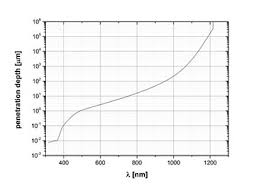The image above represents depth of penetration.

To compute for depth of penetration, two essential parameters are needed and these parameters are Conductivity (σ) and frequency (f).

The formula for calculating for depth of penetration:

d = 503.8 / √(σf)

Where:

d = Depth of Penetration
σ = Conductivity
f = Frequency

Let’s solve an example;
Find the depth of penetration when the conductivity is 12 and the frequency is 24.

This implies that;

σ = Conductivity = 12
f = Frequency = 24

d = 503.8 / √(σf)
d = 503.8 / √(12(24))
d = 503.8 / √(288)
d = 503.8 / 16.97
d = 29.68

Therefore, the depth of penetration is 29.68.

Calculating the Conductivity when the Depth of Penetration and the Frequency is Given.

σ = (503.8 / d)2 / f

Where;

σ = Conductivity
d = Depth of Penetration
f = Frequency

Let’s solve an example;
Find the conductivity when the depth of penetration is 20 and the frequency is 15.

This implies that;

d = Depth of Penetration = 20
f = Frequency = 15

σ = (503.8 / d)2 / f
σ = (503.8 / 20)2 / 15
σ = (25.19)2 / 15
σ = 634.53 / 15
σ = 42.30

Therefore, the conductivity is 42.30.

Calculating the Frequency when the Depth of Penetration and the Conductivity is Given.

f = (503.8 / d)2 / σ

Where;

f = Frequency
d = Depth of Penetration
σ = Conductivity

Let’s solve an example;
Find the frequency when the depth of penetration is 32 and the conductivity is 10.

This implies that;

d = Depth of Penetration = 32
σ = Conductivity = 10

f = (503.8 / d)2 / σ
f = (503.8 / 32)2 / 10
f = (15.74)2 / 10
f = 247.8 / 10
f = 24.78

Therefore, the frequency is 24.78.

Nickzom Calculator – The Calculator Encyclopedia is capable of calculating the depth of penetration.

To get the answer and workings of the depth of penetration using the Nickzom Calculator – The Calculator Encyclopedia. First, you need to obtain the app.

You can get this app via any of these means:

To get access to the professional version via web, you need to register and subscribe for NGN 1,500 per annum to have utter access to all functionalities.
You can also try the demo version via https://www.nickzom.org/calculator

Apple (Paid) – https://itunes.apple.com/us/app/nickzom-calculator/id1331162702?mt=8
Once, you have obtained the calculator encyclopedia app, proceed to the Calculator Map, then click on Geology under Add-on.Now, Click on Electromagnetic Method under GeologyNow, Click on Depth of Penetration under Electromagnetic Method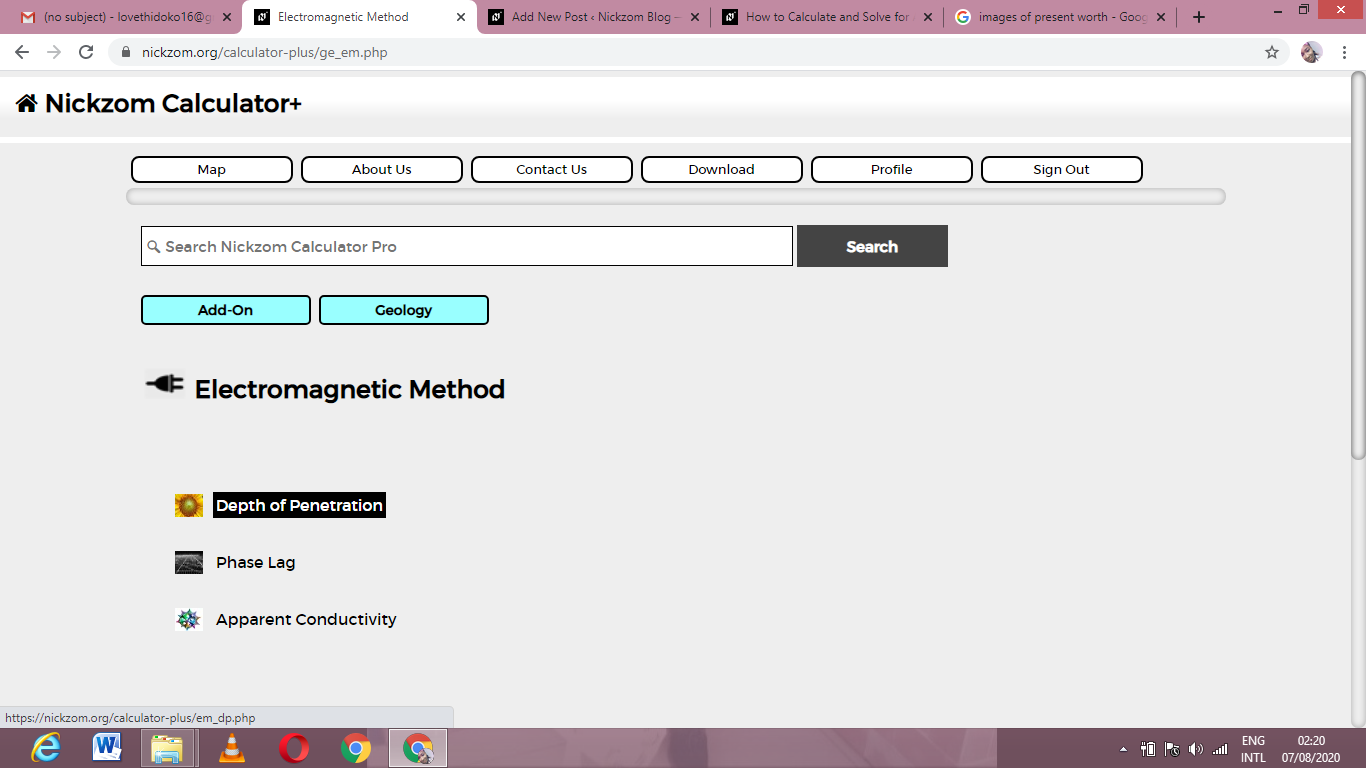The screenshot below displays the page or activity to enter your values, to get the answer for the depth of penetration according to the respective parameters which are the Conductivity (σ) and frequency (f).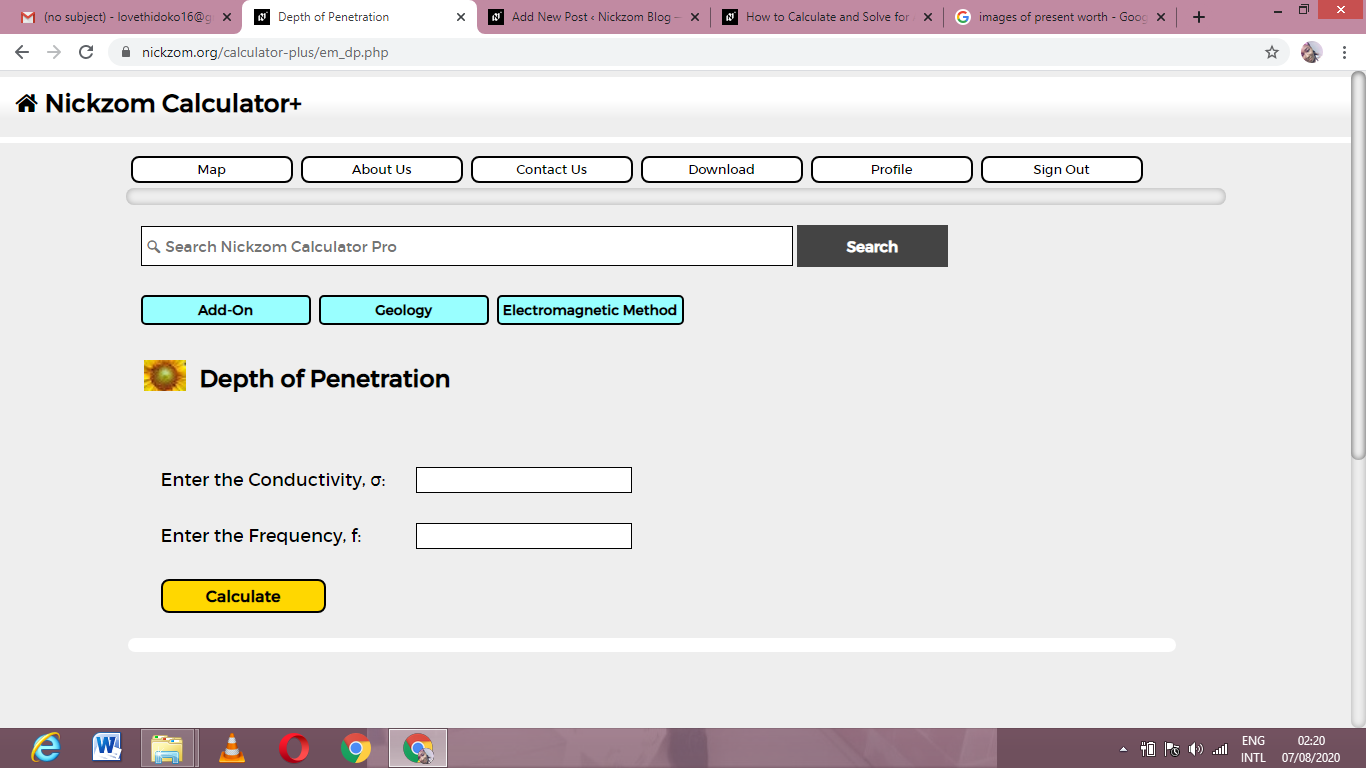Now, enter the values appropriately and accordingly for the parameters as required by the Conductivity (σ) is 12 and frequency (f) is 24.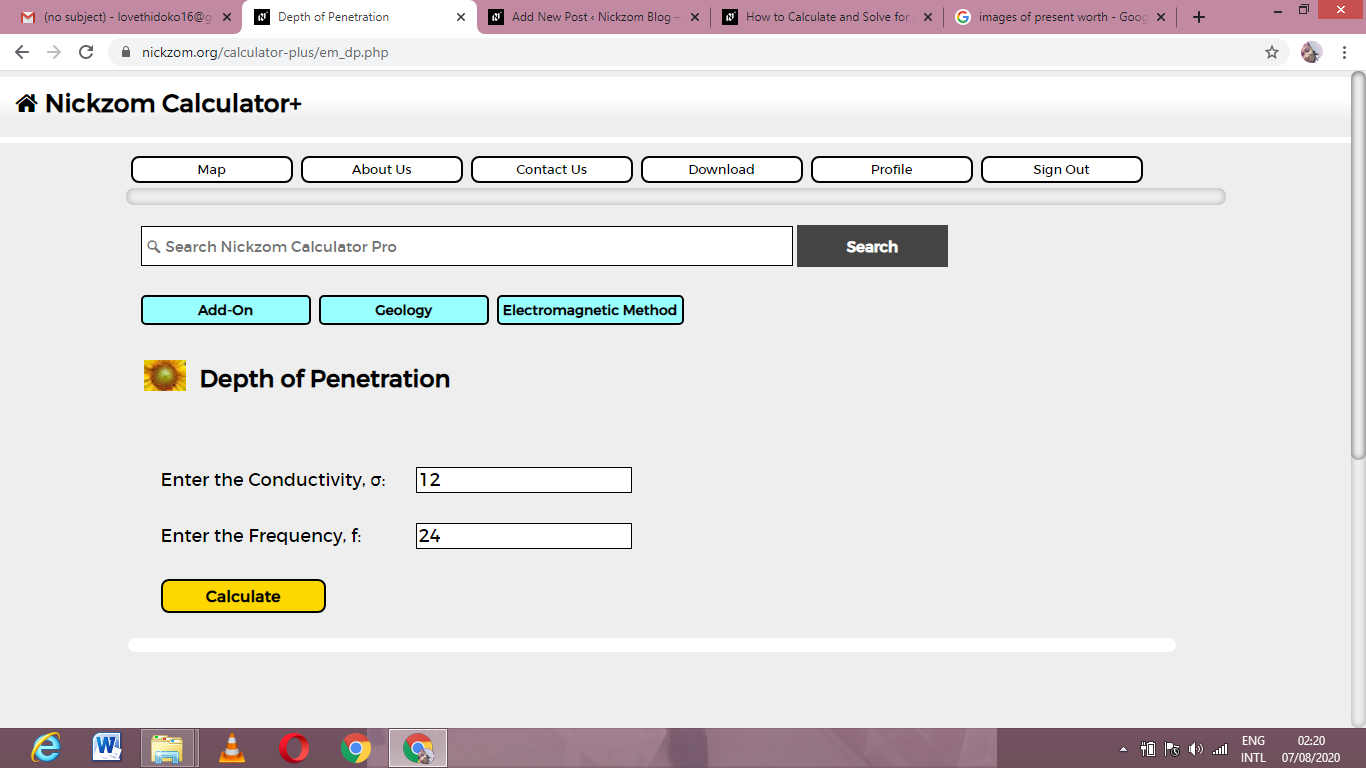Finally, Click on Calculate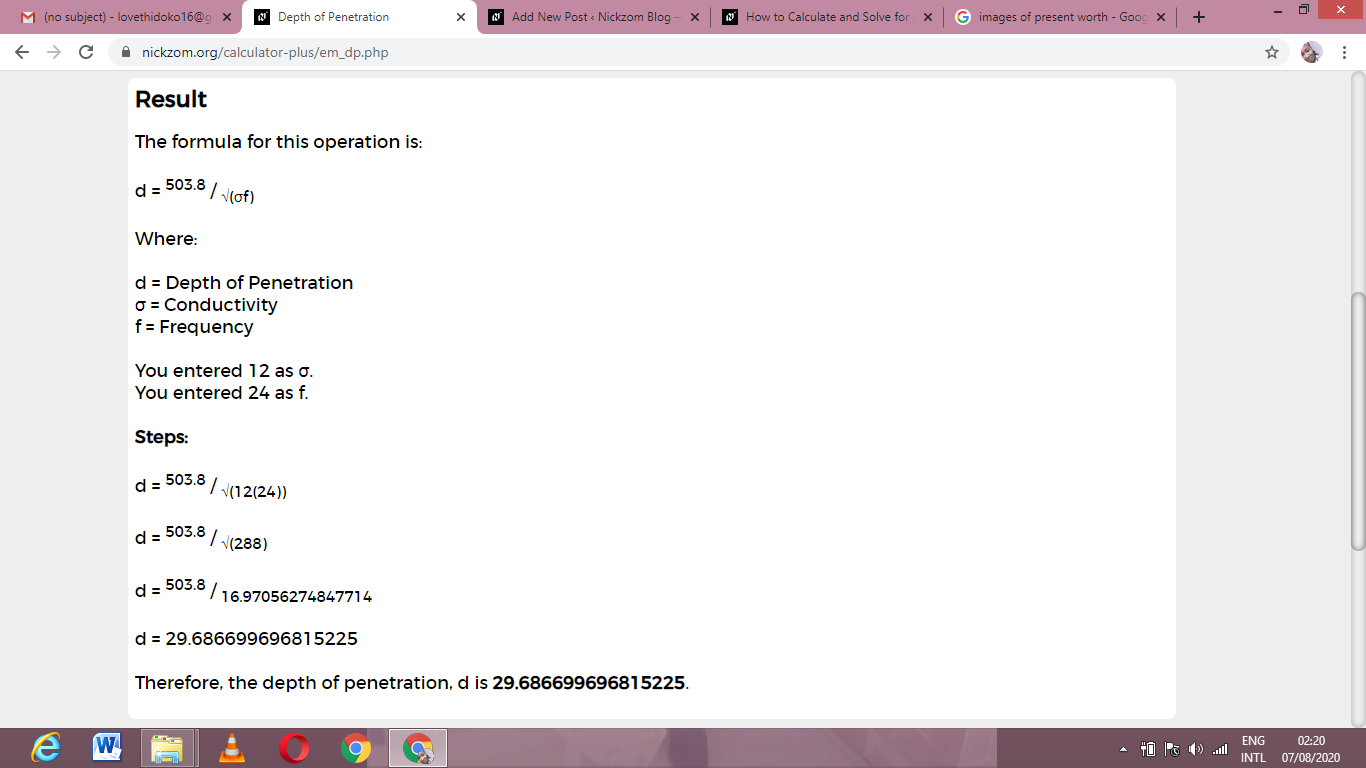As you can see from the screenshot above, Nickzom Calculator– The Calculator Encyclopedia solves for the depth of penetration and presents the formula, workings and steps too.